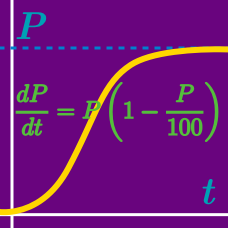Calculus

# Differential Equations - Formulate a Statement

Suppose that we drop a stone, which falls freely with no air resistance. Experiments show that, under that assumption of negligible air resistance, the acceleration ${y}^{\prime\prime}=\frac { {d}^{2}y }{ {d}^{2}t }$ of this motion is constant, i.e. equal to the so-called acceleration of gravity $g=9.8 \text{ m/sec}^2.$ State this as an ordinary differential equation for $y(t),$ the distance fallen as a function of time $t.$

Because of limited food and space, a squirrel population cannot exceed $2900$ squirrels. The population grows at a rate proportional to the product of the existing population and the attainable additional population. If $P$ denotes the squirrel population at time $t,$ which of the following equations represents the population growth rate for $k>0?$

In psychology, a stimulus-response situation is a situation in which the response $y=f(x)$ changes at a rate inversely proportional to the strength of the stimulus $x$. Which of the following equations represents this?

For a body moving along a straight line, let $y(t)$ denote its distance from a fixed point $O$ at time $t.$ Given that velocity plus distance is equal to square of time, find the ordinary differential equation of the motion.

Jack has a magic beanstalk, whose rate of growth is directly proportional to its height $H$. Which of the following equations represents his beanstalk's height as a function of time $t$?

×